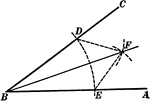### Construction Of Angle Bisector

Illustration showing how to construct the bisector of an angle.### Bisected Angle

Illustration to show that if an angle is bisected, and if a line drawn through the vertex perpendicular…### Bisected Angles

Illustration to show that the bisectors of two supplementary adjacent angles are perpendicular to each…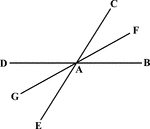### Bisected Angle

Illustration to show that the bisector of one of two vertical angles bisects the other.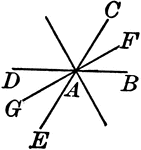### Bisected Angles

Illustration to show that the bisector of two pairs of vertical angles formed by two intersecting lines…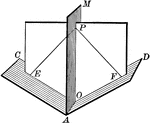### Dihedral Angle With Bisector

Illustration of a dihedral angle bisected by a plane. "Every point in a plane which bisects a dihedral…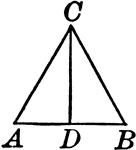### Bisected Vertical Angle of an Isosceles Triangle

Illustration to show that the bisector of the vertical angle of an isosceles triangle bisects the base,…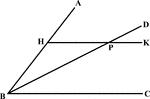### Angle Bisector Drawn Parallel to A Side

Illustration to show that if through any point in the bisector of an angle a line is drawn parallel…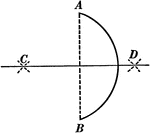### Bisecting an Arc

Illustration used to show how to bisect a given arc.### Circle With Diameter and Chord

Illustration of a circle with diameter and chord drawn. A diameter perpendicular to a chord bisects…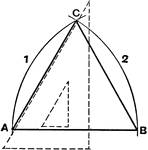### Construct Isosceles Triangle

Method to construct an isosceles triangle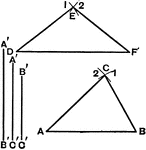### Construct Scalene Triangle

Method to construct a scalene triangle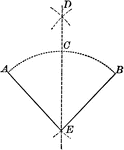### Construction of Bisecting a Given Angle

Illustration of the construction used to bisect a given angle.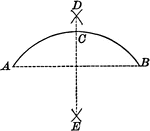### Construction of Bisecting a Given Arc

Illustration of the construction used to bisect a given arc.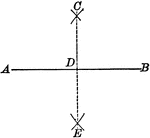### Construction of Bisecting a Given Line

Illustration of the construction used to bisect a given line.### Construction of Escribed Circles With Ex-centres

Illustration of the construction used to escribe circles with centres (centers) called ex-centres of…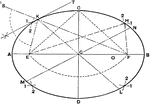### Ellipse First Method

Draftsman's first method for creating an ellipse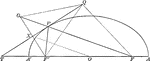### Line Bisecting Angle Between Focal Radii on Ellipse

Illustration of half of an ellipse. "If through a point P of an ellipse a line is drawn bisecting the…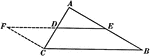### Line Joining Midpoints on a Triangle

Illustration used to prove "The line joining the mid-points of two sides of a triangle is parallel to…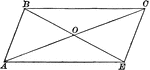### Parallelogram With Diagonals Bisecting Each Other

Illustration of a parallelogram with diagonals drawn to show they bisect each other.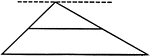### Line Bisecting Side of a Triangle

Illustration used to prove "The line bisecting one side of a triangle and parallel to another side bisects…### Bisector of an Exterior Angle of an Isosceles Triangle

Illustration to show that the bisector of an exterior angle of an isosceles triangle, formed by producing…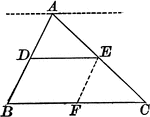### Triangle With Bisector Parallel to the Base

Illustration of a triangle showing that when a line parallel to the base of a triangle bisects one side,…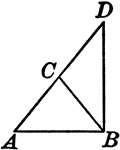### Leg Produced through the Vertex of an Isosceles Triangle

Illustration to show that if one of the legs of an isosceles triangle is produced through the vertex…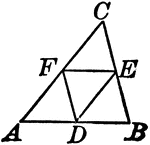### Midpoints of Triangle Divide Triangle into Four Equal Triangles

Illustration to show that if the lines joining the middle points of the sides of a triangle divide the…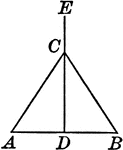### Perpendicular Bisector of the Base of an Isosceles Triangle

Illustration to show that the perpendicular bisector of the base of an isosceles triangle passes through…